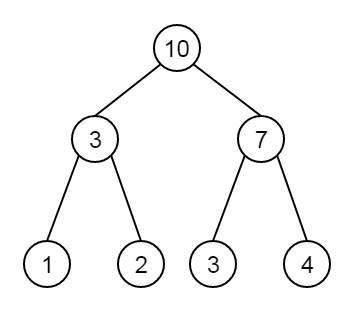# Find sum of all nodes of the given perfect binary tree in C++

C++Server Side ProgrammingProgramming

Suppose we have a positive integer L, which represents the number of levels in a perfect binary tree. The leaf nodes in this perfect binary tree are numbered starting from 1 to n. Where the n is number of leaf nodes. The parent node is the sum of children. Our task is to write a program to print the sum of all of the nodes of this perfect binary tree. So if the tree is like below −So total sum is 30.

If we see closer, we need to find the sum of all of the nodes. As the leaf nodes are holding values from 1 to n, then we can use the formula n(n+1)/2 to get sum of leaf nodes. As this is perfect binary tree, the sum of each levels will be same. So find the sum of last level, then multiply it with number of levels.

## Example

Live Demo

#include<iostream>
#include<cmath>
using namespace std;
int treeSum(int level) {
int total_leaves = pow(2, level - 1);
int leaf_sum = 0;
leaf_sum = (total_leaves * (total_leaves + 1)) / 2;
int sum = leaf_sum * level;
return sum;
}
int main() {
int levels = 4;
cout << "Sum of all nodes for a perfect binary tree with level " << levels << " is: " << treeSum(levels);
}

## Output

Sum of all nodes for a perfect binary tree with level 4 is: 144